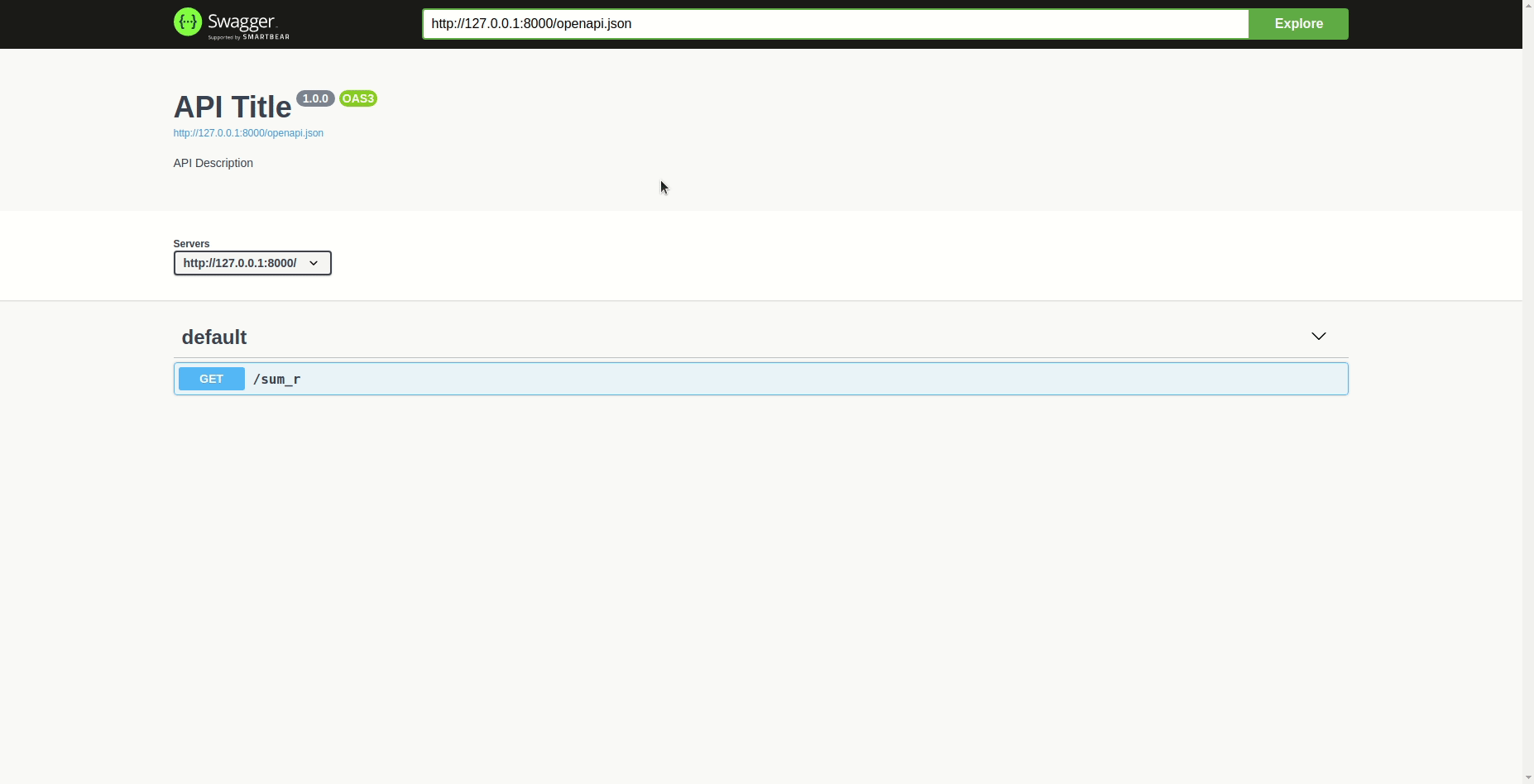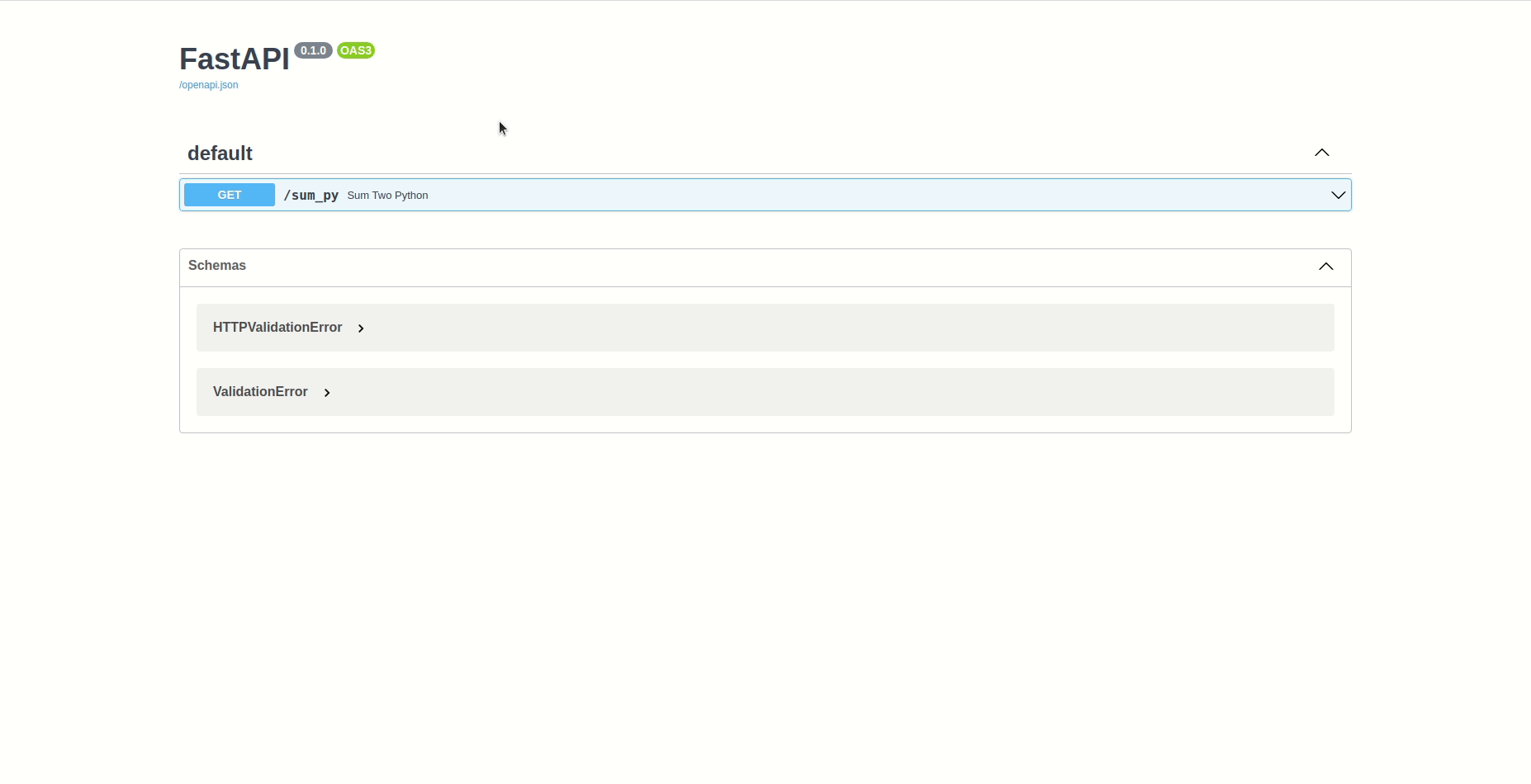# Why not both?

By Adson Costanzi Filho | July 13, 2021

## Motivation

I believe the most common discussion on data science teams is R vs Python. I saw myself in some of these discussions a couple of times and my position is always “Why not use both?”. The idea of this post is not to compare R vs Python but to show how easy it is to integrate both languages using APIs (LIKE A PRO), this way we can use the best of each. Also, the post won’t focus on the deployment and structure needed to bring the APIs online, instead, I will demonstrate it locally (to make it simple).

This post is divided into 3 sections: Creating a function, Creating an API, and Calling an API. In the first section, you will find a simple function created in R and Python, the second section is about how to transform that functions into APIs, the last one is how we can call/use the APIs.

## Creating a function

Imagine that you are working in a data science team and someone needs a piece of code that return the sum of two values (sorry for the stupid example). See, the request is not about R, Python, Julia, Java, or whatever, but to solve the problem! So, let’s solve the problem the best way we can, and let’s write a function to solve it in R and Python.

This post will not cover the guidelines to create useful functions. For that I recommend you to take a look at the Functions section of the Advanced R Book.

### Function in R

A simple function to return the sum of two values in R would look like this:

``````sum_two_r <- function(x, y)
{
result <- x + y
return(result)
}``````

It is done, now we can call it inside R like this:

``sum_two_r(x = 1, y = 1)``
``##  2``

### Function in Python

A simple function to return the sum of two values in Python would look like this:

``````def sum_two_python(x, y):
result = x + y
return result``````

It is done, now we can call it inside Python like this:

``sum_two_python(x = 1, y = 1)``
``## 2``

## Creating an API

The problem is almost solved, but we still need to find the best way to share our solution with the rest of the team. Well, the part of the team that works in R can use the function we wrote in R, but that function is simply not available to the part of the team that uses Python, the same happens with the code written in Python which is simply not available to anyone using R. So, it is necessary to create a solution independent of the language to make it reachable for everyone on the team.

I know the reticulate package can help, but it’s a one-way solution, and we are interested in a more generic form of integration that can be extended not just to Python, but to any programming language.

One good solution would be to create and deploy an API with our piece of code, this way the rest of the team can interact with it independently, in other words, doesn’t matter anymore what was the language you used to solve the problem. To do that we are going to use the plumber package for R and the FastAPI package for Python.

This post will not cover how to deploy the APIs. However, if you are interested in learning how to bring your APIs online you can use this link for plumber, and this link for FastAPI.

### API in R

The first step to create an API in R is to install the `plumber` package:

``install.packages("plumber")``

That done, let’s get back to our function file and add a couple of things to it.

``````#* @param x first number
#* @param y second number
#* @get /sum_r

sum_two_r <- function(x, y)
{
result <- as.numeric(x) + as.numeric(y)
return(result)
}``````

Notice that we add some “comments” at the begging of the code very similar to the ones used in the `roxygen2` package. These comments are the key that plumber uses to transform your functions in APIs endpoints. Also, for this example, we defined a GET request, the same could be done, for example, with POST requests by changing the comment to `#* @post /sum_r`.

If you are not familiar with HTTP requests you can take a look at this link.

You can see that it was included the `as.numeric()` transformation, which is necessary because the parameters “enter” R as strings, and to apply mathematical operations it is necessary to transform the numeric ones into numbers.

The next step is to deploy it! To bring it online you just need to provide the file path and the port you want to expose the API. We are going to make it available locally (localhost) at the port 8000 by running:

``````library(magrittr)
library(plumber)

pr('functions/sum_r.R') %>%
pr_run(port = 8000)``````

Done! Now our API is exposed and you can access the Swagger documentation at http://localhost:8000/__docs__/, and you can interact with it by pressing GET, followed by the Try it out button, fill in the parameters and press Execute.### API in Python

The first step to create an API in Python is to install the `FastAPI` package. On the terminal run:

``````\$ pip install fastapi[all]
``````

That done, let’s get back to our function file and add a couple of things to it.

``````from fastapi import FastAPI

app = FastAPI()

@app.get("/sum_py")
def sum_two_python(x:float, y:float):
result = x + y
return result``````

Notice that we import the fastapi package and we created an object called app that is a FastAPI instance. Then we defined it as a GET request, the same could be done, for example, with POST requests by changing to `@app.post("/sum_py")`.

You can see that it was included the `:float` to force the variable type to be numeric, that is necessary because the default APIs calls “enter” Python as strings, and to apply mathematical operations it is necessary to transform the numeric ones into numbers.

The next step is to deploy it! To bring it online you just need to open the terminal and get inside the folder you saved your Python API script. I named my file as “sum_py” and I am going to make it available locally (localhost) at the port 8080 by running:

``````\$ uvicorn sum_py:app --port 8080 --reload
``````

The reload option will reload your API every time you save the file. It is a very good feature for development!

Done! Now our API is exposed and you can access the Swagger documentation at http://localhost:8080/docs#/, and you can interact with it by pressing GET, followed by the Try it out button, fill in the parameters and press Execute.Of course, in the real world, the deployment of your APIs shouldn’t be local, but the logic will be (almost) the same. Also, there are MUCH MORE FEATURES on the API development, so my advice would be to read the plumber and FastAPI documentation to learn more about it.

## Calling an API

From now on, the solution should be available for EVERYONE in the team, whether they use Python, R, etc. Now things will get crazy because we are going to call the Python API in R and the R API in Python!!! 😵

PS: Keep both APIs running locally!

### Calling an API in R

It is very simple to call APIs using R, and for that, we are going to use the `httr` package. Let’s install it:

``install.packages("httr")``

Do you remember that our Python API is running locally (localhost) at the port 8080? Well, that and the request type (GET, in this case) are the only information we need to call it through R.

``````library(httr)

python_request <- GET('http://localhost:8080/sum_py?x=1&y=1')

python_result <- content(python_request)

python_result``````
``##  2``

### Calling an API in Python

Let’s do the same, but this time we are going to call the R API through Python! For that, it will be necessary to install the `requests` package.

``````\$ pip install requests
``````

Our R API is also running on localhost but at the port 8000. Again, that and the request type (GET, in this case) are the only information we need to call it through Python.

``````import requests

r_request = requests.get("http://localhost:8000/sum_r?x=1&y=1")

print(r_request.json())``````
``## ``

## That is all

There are MUCH MORE to discuss when we talk about APIs/deploy/calls/requests, but the idea was to make it simple, to demonstrate the possibilities, and to STOP THE FIGHT between R and Python 😆. I believe this post is a good example of how to integrate both languages (LIKE A PRO), because, in the end, we want to SOLVE THE PROBLEM!!! Does it really matter if you solved it in Python or R?

I hope someone finds this useful. As always your feedback is much appreciated, feel free to get in touch with me over social media! 😄